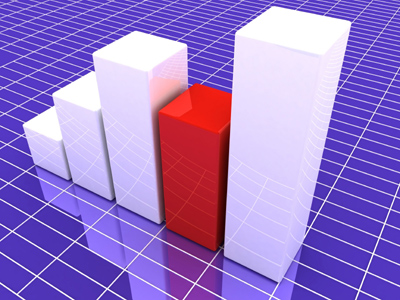Graphs are useful for recording data.

# Algebra - Graphs 4

This Math quiz is called 'Algebra - Graphs 4' and it has been written by teachers to help you if you are studying the subject at middle school. Playing educational quizzes is a fabulous way to learn if you are in the 6th, 7th or 8th grade - aged 11 to 14.

It costs only \$12.50 per month to play this quiz and over 3,500 others that help you with your school work. You can subscribe on the page at Join Us

When it comes to graphs, you'll need to know an exponent from a coordinate. Fed up of algebra? Sick of graphs? We have some great news for you! This is the last Math quiz in this series on graphs. It tests more complicated aspects of the world of graphs.

Go on, give it a whirl and see how much you have learned so far.

1.
Which of these graphs would give you the most shallow gradient?
y = 3x - 1
y = 4x - 1
y = 5x - 1
y = 6x -1
2.
If a graph equation contains only the signs of plus, minus and equals then what could you definitely say about the line?
It is very long
It is very short
It is straight
It is curved
3.
Which of these equations would give the steepest gradient?
y = x
y = 2x
y = 3x
y = 4x
In the correct answer, each movement of a unit along the x axis means a movement of 4 units up the y axis
4.
On a distance-time graph what does a line segment that is horizontal tell you?
The data is incorrect
The distance traveled was slowing down
The distance traveled was speeding up
There was no movement during the period
5.
Two coordinates of a line are as follows: (2, 15) and (6, 10). How might the line best be described?
It is horizontal
It is vertical
It is worth remembering that you can have both positive and negative gradients in ANY quadrant
6.
Which of these equations would give the steepest gradient?
y = x
y = x + 1
y = x + 2
All the above are the same gradient
If all the lines were plotted on the same graph they would run parallel to each other
7.
Two coordinates of a line are as follows: (3, 12) and (2, 8). What is the equation?
y = 2x
y = 4x
y = 6x
y = 8x
If you plot the coordinates you will see that for every unit moved along the x axis, you move 4 units up the y axis. Working out an equation in this way has the grand name of the 'gradient-intercept' method
8.
What is the mid point of the line (1, 9) (5, 15)
(1, 15)
(3, 12)
(6, 24)
(9, 15)
Take the first number in the first bracket and add it to the first number in the second bracket and divide the answer by two - gives you 3. Then do the same for the second numbers in the brackets and you will get 12
9.
If a graph equation contains an exponent then what could you definitely say about the line?
It is very long
It is very short
It is straight
It is curved
Remember an exponent is the small number to the upper-right of another number. As soon as you see one of these in an equation you should expect a curve
10.
Two coordinates of a line are as follows: (3, 21) and (2, 14). How might the line best be described?